### IMO Shortlist 2012 problem A2

Kvaliteta:
Avg: 0,0
Težina:
Avg: 6,0
Let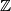$\mathbb{Z}$ and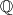$\mathbb{Q}$ be the sets of integers and rationals respectively.
a) Does there exist a partition of$\mathbb{Z}$ into three non-empty subsets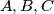$A,B,C$ such that the sets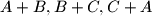$A+B, B+C, C+A$ are disjoint?
b) Does there exist a partition of$\mathbb{Q}$ into three non-empty subsets$A,B,C$ such that the sets$A+B, B+C, C+A$ are disjoint?

Here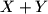$X+Y$ denotes the set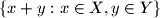$\{ x+y : x \in X, y \in Y \}$, for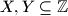$X,Y \subseteq \mathbb{Z}$ and for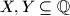$X,Y \subseteq \mathbb{Q}$.
Izvor: Međunarodna matematička olimpijada, shortlist 2012